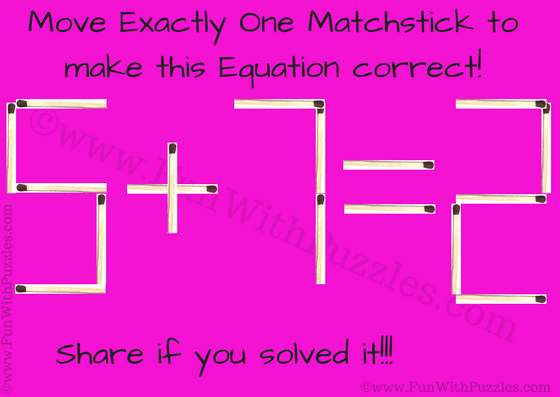Here is an easy Matchstick Math Brain Teaser for Kids. In this you are given a simple number equation that reads 5+7=2. This equation is made with matchsticks. Your challenge is moving just one matchstick to another position and making this equation correct. Let's see how much time it will take to solve this easy Matchstick Math Brain Teaser?Can you solve this 5+7=2 Matchstick Puzzle?

The answer to this "Matchstick Math Brain Teaser for Kids", can be viewed by clicking on the button. Please do give your best try before looking at the answer.

#### 1 comment:

Anonymous said...

5-7=-2